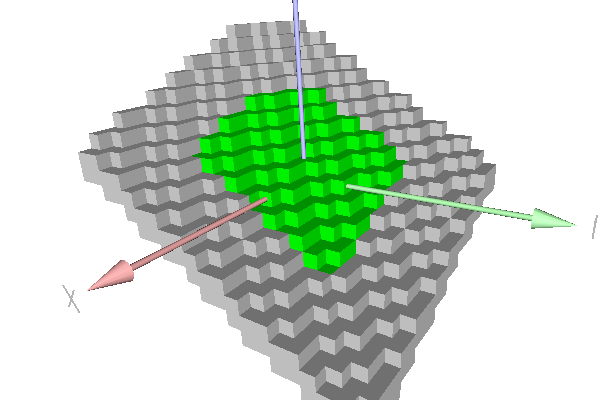DGtal  1.3.beta
io/viewers/viewer3D-7-stdplane.cpp

This example shows the recognition of a simple standard digital plane with the COBA algorithm (class DGtal::COBAGenericStandardPlaneComputer). Green points belong to the naive plane. Grey points show farther points that also belong to the strip.

Standard plane recognition with COBA algorithm
\$ ./examples/io/viewers/viewer3D-7-stdplanePiece of standard plane.
#include <cstdlib>
#include <iostream>
#include "DGtal/base/Common.h"
#include "DGtal/helpers/StdDefs.h"
#include "DGtal/io/viewers/Viewer3D.h"
#include "DGtal/geometry/surfaces/COBAGenericStandardPlaneComputer.h"
using namespace std;
using namespace DGtal;
// Standard services - public :
/*
Displays in the \a viewer all the points in the \a domain that
satisfies the predicate \a pred.
*/
template <typename Viewer3D, typename Domain, typename Predicate>
void
const Domain & domain, const Predicate & pred )
{
for ( typename Domain::ConstIterator itB = domain.begin(), itE = domain.end();
itB != itE; ++itB )
{
if ( pred( *itB ) )
viewer << *itB;
}
}
/*
Displays in the \a viewer all the points in the range [it,itE).
*/
template <typename Viewer3D, typename InputIterator>
void
displayRange( Viewer3D & viewer, InputIterator it, InputIterator itE )
{
for ( ; it != itE; ++it )
viewer << *it;
}
/*
Returns all the points in the \a domain that belongs to the standard
plane mu <= a*x+b*y+c*z < mu+|a|+|b|+|c|.
*/
template <typename Domain>
std::vector<typename Domain::Point> pointsInStandardPlane
( const Domain & domain,
typename Domain::Integer a,
typename Domain::Integer b,
typename Domain::Integer c,
typename Domain::Integer mu )
{
typedef typename Domain::Integer Integer;
typedef typename Domain::Point Point;
std::vector<Point> pts;
Integer mup = mu + abs(a) + abs(b) + abs(c);
for ( ConstIterator it = domain.begin(), itE = domain.end();
it != itE; ++it )
{
Point p = *it;
Integer r = a * p[ 0 ] + b * p[ 1 ] + c * p[ 2 ];
if ( ( mu <= r ) && ( r < mup ) )
pts.push_back( p );
}
return pts;
}
int main( int argc, char** argv )
{
using namespace Z3i;
unsigned int nb = 0;
unsigned int nbok = 0;
QApplication application(argc,argv);
unsigned int diameter = argc > 1 ? atoi( argv[ 1 ] ) : 10;
int a = argc > 2 ? atoi( argv[ 2 ] ) : 2;
int b = argc > 3 ? atoi( argv[ 3 ] ) : 3;
int c = argc > 4 ? atoi( argv[ 4 ] ) : 5;
int mu = argc > 5 ? atoi( argv[ 5 ] ) : 0;
trace.beginBlock ( "Testing class COBAGenericStandardPlaneComputer" );
trace.info() << "Recognizing plane "
<< mu << " <= " << a << " * x + "
<< b << " * y + " << c << " * z < "
<< (mu+abs(a)+abs(b)+abs(c)) << std::endl;
Domain domain1( Point( -diameter, -diameter, -diameter ),
Point( diameter, diameter, diameter ) );
typedef PlaneComputer::Primitive Primitive;
PlaneComputer plane;
plane.init( 2*diameter, 1, 1 );
std::vector<Point> recognized = pointsInStandardPlane( domain1,
a, b , c, mu );
++nb, nbok += plane.extend( recognized.begin(), recognized.end() ) ? 1 : 0;
trace.info() << "(" << nbok << "/" << nb
<< ") All points are recognized." << std::endl;
trace.info() << " - Plane=" << plane
<< std::endl;
Primitive strip = plane.primitive();
trace.info() << "strip=" << strip
<< " axis=" << strip.mainAxis()
<< " axiswidth=" << strip.axisWidth()
<< " diag=" << strip.mainDiagonal()
<< " diagwidth=" << strip.diagonalWidth()
<< std::endl;
++nb, nbok += ( strip.diagonalWidth() < sqrt(3.0) ) ? 1 : 0;
trace.info() << "(" << nbok << "/" << nb
<< ") Diagonal width < sqrt(3)." << std::endl;
trace.emphase() << ( (nb == nbok) ? "Passed." : "Error." ) << endl;
MyViewer viewer;
viewer.show();
Color red( 255, 0, 0 );
Color green( 0, 255, 0 );
Color grey( 200, 200, 200 );
Domain domain2( Point( -2*diameter, -2*diameter, -2*diameter ),
Point( 2*diameter, 2*diameter, 2*diameter ) );
viewer << CustomColors3D( red, red );
for ( std::vector<Point>::const_iterator it = recognized.begin(),
itE = recognized.end(); it != itE; ++it )
if ( ! strip( *it ) ) viewer << *it;
viewer << CustomColors3D( green, green );
displayRange( viewer, plane.begin(), plane.end() );
viewer << CustomColors3D( grey, grey );
displayPredicate( viewer, domain2, strip );
trace.info() << "- Points in green have been recognized as belonging to this standard plane." << std::endl;
trace.info() << "- Points in grey belongs also to the parallel strip of the recognized standard plane." << std::endl;
trace.info() << "- Points in red belongs to the parallel strip of the recognized standard plane but not to the input standard plane: NONE should be red." << std::endl;
return application.exec();
}
// //
displayRange
void displayRange(const R &r)
ConstIterator
MyDigitalSurface::ConstIterator ConstIterator
Definition: greedy-plane-segmentation-ex2.cpp:93
DGtal::HyperRectDomain< Space >
DGtal::Trace::endBlock
double endBlock()
DGtal::Color
Structure representing an RGB triple with alpha component.
Definition: Color.h:67
pointsInStandardPlane
std::vector< typename Domain::Point > pointsInStandardPlane(const Domain &domain, typename Domain::Integer a, typename Domain::Integer b, typename Domain::Integer c, typename Domain::Integer mu)
Definition: testChordGenericStandardPlaneComputer.cpp:60
DGtal::Trace::emphase
std::ostream & emphase()
DGtal::trace
Trace trace
Definition: Common.h:154
displayPredicate
void displayPredicate(Viewer3D &viewer, const Domain &domain, const Predicate &pred)
Definition: viewer3D-7-planes.cpp:64
DGtal::Display3D::updateDisplay
@ updateDisplay
Definition: Display3D.h:249
DGtal::Trace::beginBlock
void beginBlock(const std::string &keyword="")
DGtal::Trace::info
std::ostream & info()
DGtal::Viewer3D
Definition: Viewer3D.h:135
DGtal
DGtal is the top-level namespace which contains all DGtal functions and types.
DGtal::HyperRectDomain::end
const ConstIterator & end() const
Definition: HyperRectDomain.h:201
main
int main(int argc, char **argv)
Definition: testArithmeticDSS-benchmark.cpp:147
DGtal::COBAGenericStandardPlaneComputer::init
void init(InternalInteger diameter, InternalInteger widthNumerator=NumberTraits< InternalInteger >::ONE, InternalInteger widthDenominator=NumberTraits< InternalInteger >::ONE)
Integer
Point::Coordinate Integer
DGtal::functions::abs
T abs(const T &a)
Definition: BasicMathFunctions.h:116
DGtal::HyperRectDomain_Iterator
Iterator for HyperRectDomain.
Definition: HyperRectDomain_Iterator.h:142
domain
Domain domain
Definition: testProjection.cpp:88
DGtal::HyperRectDomain< Space >::Integer
Space::Integer Integer
Definition: HyperRectDomain.h:114
DGtal::PointVector< dim, Integer >
DGtal::Viewer3D::show
virtual void show()
Overload QWidget method in order to add a call to updateList() method (to ensure that the lists are w...
DGtal::HyperRectDomain::begin
const ConstIterator & begin() const
Definition: HyperRectDomain.h:176
Point
MyPointD Point
Definition: testClone2.cpp:383
DGtal::COBAGenericStandardPlaneComputer
Aim: A class that recognizes pieces of digital planes of given axis width. When the diagonal width is...
Definition: COBAGenericStandardPlaneComputer.h:128